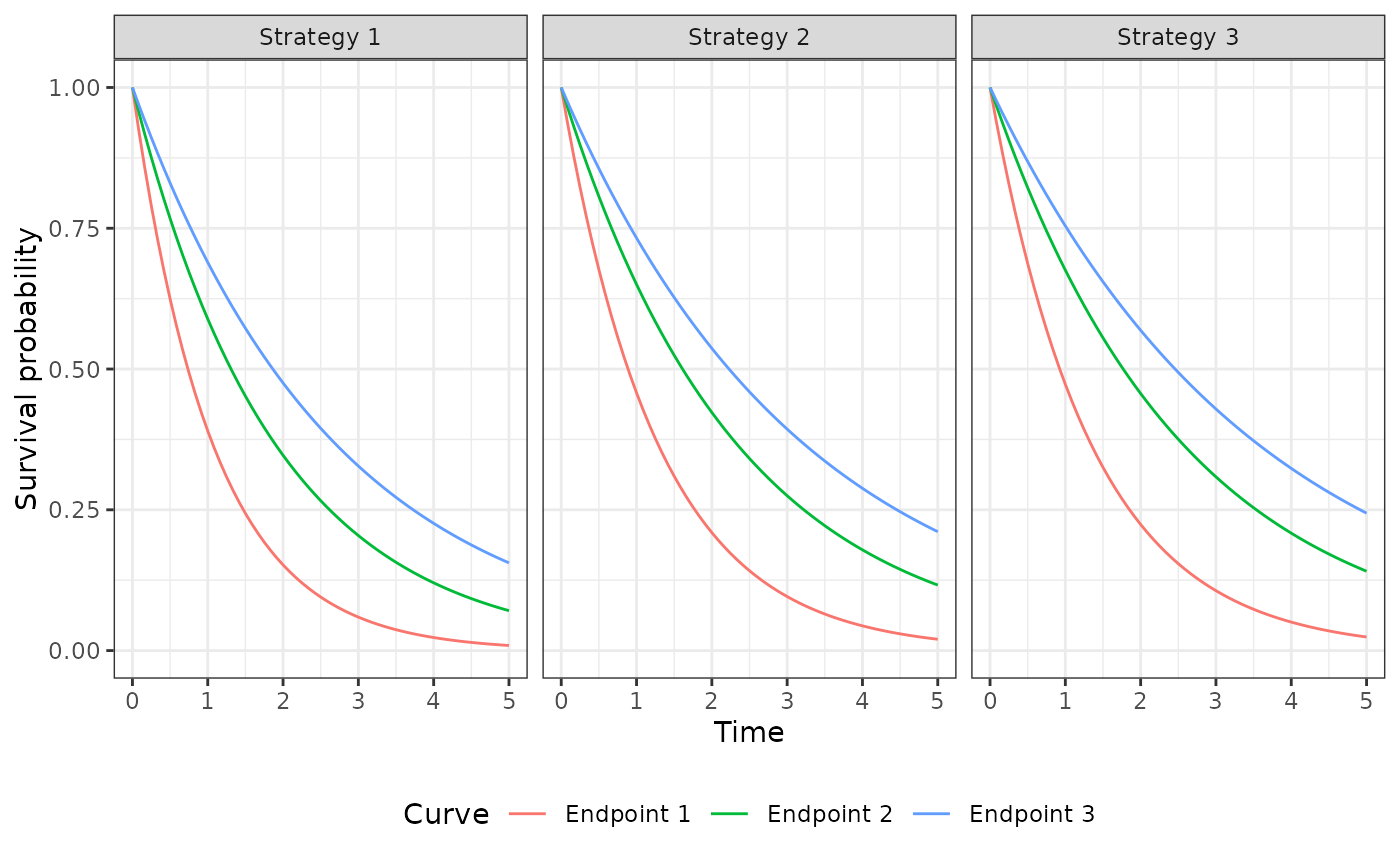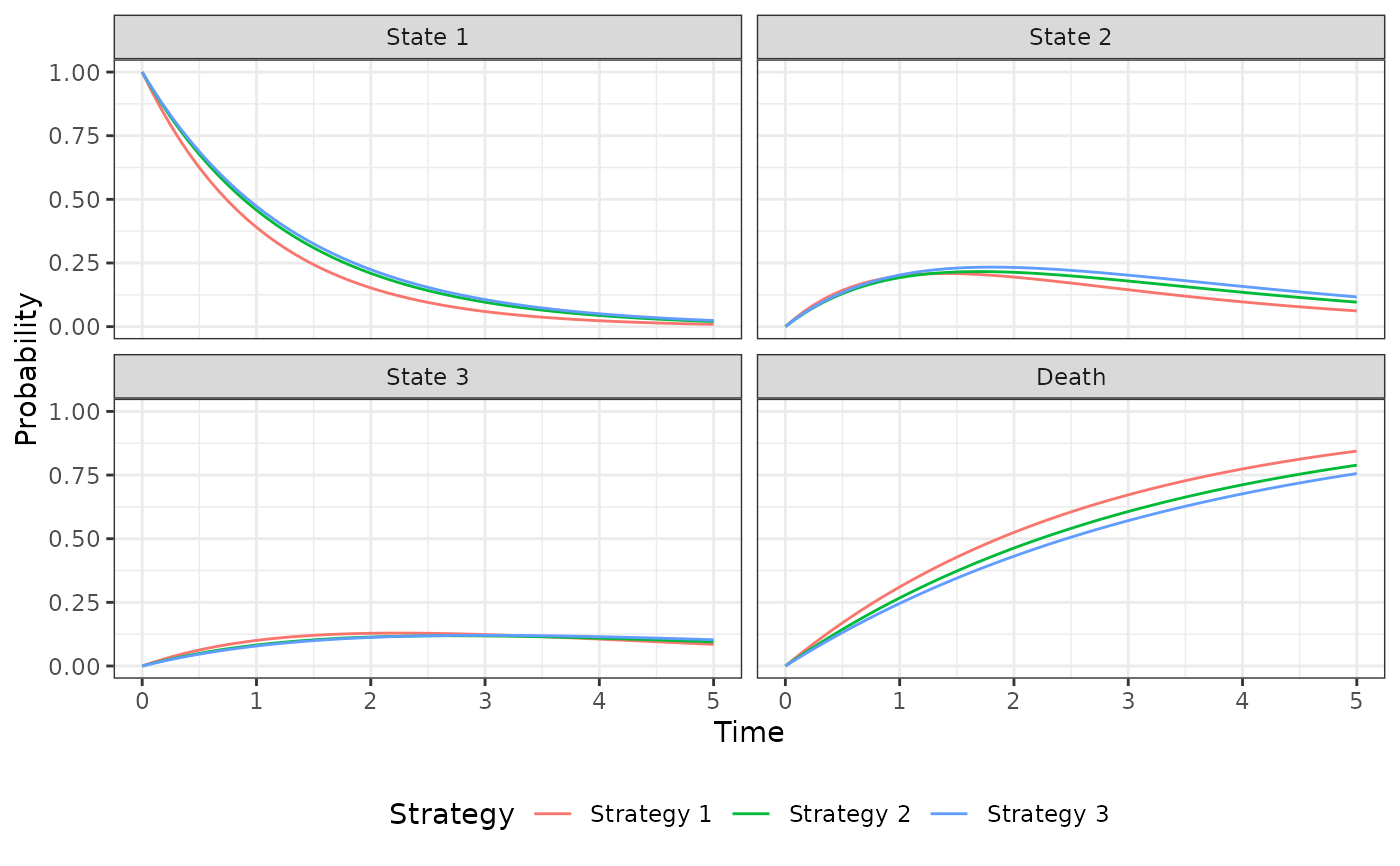Simulate outcomes from an N-state partitioned survival model.

## Format

An R6::R6Class object.

The PsmCurves documentation describes the class for the survival models and the StateVals documentation describes the class for the cost and utility models. A PsmCurves object is typically created using create_PsmCurves(). The PsmCurves documentation provides an example in which the model is parameterized from parameter objects (i.e., without having the patient-level data required to fit a model with R). A longer example is provided in vignette("psm").

## Public fields

survival_models

The survival models used to predict survival curves. Must be an object of class PsmCurves.

utility_model

The model for health state utility. Must be an object of class StateVals.

cost_models

The models used to predict costs by health state. Must be a list of objects of class StateVals, where each element of the list represents a different cost category.

n_states

Number of states in the partitioned survival model.

t_

A numeric vector of times at which survival curves were predicted. Determined by the argument t in $sim_curves(). survival_ An object of class survival simulated using sim_survival(). stateprobs_ An object of class stateprobs simulated using $sim_stateprobs().

qalys_

An object of class qalys simulated using $sim_qalys(). costs_ An object of class costs simulated using $sim_costs().

## Methods

### Method new()

Create a new Psm object.

### Method sim_stateprobs()

Simulate health state probabilities from survival_ using a partitioned survival analysis.

Psm$sim_stateprobs() #### Returns An instance of self with simulated output of class stateprobs stored in stateprobs_. ### Method sim_qalys() Simulate quality-adjusted life-years (QALYs) as a function of stateprobs_ and utility_model. See sim_qalys() for details. #### Usage Psm$sim_qalys(
dr = 0.03,
integrate_method = c("trapz", "riemann_left", "riemann_right"),
lys = TRUE
)

#### Arguments

dr

Discount rate.

integrate_method

Method used to integrate state values when computing costs or QALYs. Options are trapz for the trapezoid rule, riemann_left for a left Riemann sum, and riemann_right for a right Riemann sum.

lys

If TRUE, then life-years are simulated in addition to QALYs.

#### Returns

An instance of self with simulated output of class qalys stored in qalys_.

### Method sim_costs()

Simulate costs as a function of stateprobs_ and cost_models. See sim_costs() for details.

#### Arguments

by_grp

If TRUE, then costs and QALYs are computed by subgroup. If FALSE, then costs and QALYs are aggregated across all patients (and subgroups).

### Method clone()

The objects of this class are cloneable with this method.

Psm$clone(deep = FALSE) #### Arguments deep Whether to make a deep clone. ## Examples library("flexsurv") library("ggplot2") theme_set(theme_bw()) # Model setup strategies <- data.frame(strategy_id = c(1, 2, 3), strategy_name = paste0("Strategy ", 1:3)) patients <- data.frame(patient_id = seq(1, 3), age = c(45, 50, 60), female = c(0, 0, 1)) states <- data.frame(state_id = seq(1, 3), state_name = paste0("State ", seq(1, 3))) hesim_dat <- hesim_data(strategies = strategies, patients = patients, states = states) labs <- c( get_labels(hesim_dat), list(curve = c("Endpoint 1" = 1, "Endpoint 2" = 2, "Endpoint 3" = 3)) ) n_samples <- 2 # Survival models surv_est_data <- psm4_exdata$survival
fit1 <- flexsurvreg(Surv(endpoint1_time, endpoint1_status) ~ factor(strategy_id),
data = surv_est_data, dist = "exp")
fit2 <- flexsurvreg(Surv(endpoint2_time, endpoint2_status) ~ factor(strategy_id),
data = surv_est_data, dist = "exp")
fit3 <- flexsurvreg(Surv(endpoint3_time, endpoint3_status) ~ factor(strategy_id),
data = surv_est_data, dist = "exp")
fits <- flexsurvreg_list(fit1, fit2, fit3)

surv_input_data <- expand(hesim_dat, by = c("strategies", "patients"))
psm_curves <- create_PsmCurves(fits, input_data = surv_input_data,
uncertainty = "bootstrap", est_data = surv_est_data,
n = n_samples)

# Cost model(s)
cost_input_data <- expand(hesim_dat, by = c("strategies", "patients", "states"))
fit_costs_medical <- lm(costs ~ female + state_name,
data = psm4_exdata$costs$medical)
psm_costs_medical <- create_StateVals(fit_costs_medical,
input_data = cost_input_data,
n = n_samples)

# Utility model
utility_tbl <- stateval_tbl(tbl = data.frame(state_id = states$state_id, min = psm4_exdata$utility$lower, max = psm4_exdata$utility$upper), dist = "unif") psm_utility <- create_StateVals(utility_tbl, n = n_samples, hesim_data = hesim_dat) # Partitioned survival decision model psm <- Psm$new(survival_models = psm_curves,
utility_model = psm_utility,
cost_models = list(medical = psm_costs_medical))
psm$sim_survival(t = seq(0, 5, 1/12)) autoplot(psm$survival_, labels = labs, ci = FALSE, ci_style = "ribbon")psm$sim_stateprobs() autoplot(psm$stateprobs_, labels = labs)psm$sim_costs(dr = .03) head(psm$costs_)
#>    sample strategy_id patient_id grp_id state_id   dr category    costs
#> 1:      1           1          1      1        1 0.03  medical 32040.82
#> 2:      1           1          1      1        2 0.03  medical 17241.33
#> 3:      1           1          1      1        3 0.03  medical 17165.95
#> 4:      1           1          2      1        1 0.03  medical 32040.82
#> 5:      1           1          2      1        2 0.03  medical 17241.33
#> 6:      1           1          2      1        3 0.03  medical 17165.95
head(psm$sim_qalys(dr = .03)$qalys_)
#>    sample strategy_id patient_id grp_id state_id   dr     qalys       lys
#> 1:      1           1          1      1        1 0.03 0.8759927 1.0170828
#> 2:      1           1          1      1        2 0.03 0.4895583 0.6482033
#> 3:      1           1          1      1        3 0.03 0.3468770 0.5055821
#> 4:      1           1          2      1        1 0.03 0.8759927 1.0170828
#> 5:      1           1          2      1        2 0.03 0.4895583 0.6482033
#> 6:      1           1          2      1        3 0.03 0.3468770 0.5055821